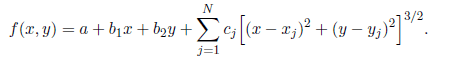# Partial derivative of radial basis function

• Fritzmit
In summary, the partial derivatives of f with respect to x and y can be calculated using the chain rule and the given equation for f. The summation and power to 3/2 can be handled by taking the partial derivative under the sum and using the chain rule multiple times.

## Homework Statement

Calculate the partial derivatives (∂f/∂x & ∂f/∂y)

## Homework Equations## The Attempt at a Solution

really confusing me with the use of the summation and power to 3/2. This is my attempt, most definitely wrong but still tried.

∂f/∂x = x + c1*(2*(x-x1))*([( x-x1 )^2 + (y-y1)^2)]^(1/2)) + ... + cN*(2*(x-xN))*([( x - xN )^2 + (y-yN)^2)]^(1/2))

∂f/∂y = y + c1*(2*(y-y1))*([( x-x1 )^2 + (y-y1)^2)]^(1/2)) + ... + cN*(2*(y-yN))*([( x - xN )^2 + (y-yN)^2)]^(1/2))

sorry, I probably should know this, but I can't really think straight right now -_-
not sure if its just double chain rule or what...

thank you for any help

looks close
$$f(x,y) = a + b_1x + b_2y + \sum_{j=1}^{N}c_j((x-x_j)^2+(y-y_j)^2)^{\frac{3}{2}}$$

there's no problem taking the partial derivative under the sum, so as you say it just becomes a chain rule question
$$f_x = \frac{\partial}{\partial x}f(x,y) = \frac{\partial}{\partial x}(a + b_1x + b_2y + \sum_{j=1}^{N}c_j((x-x_j)^2+(y-y_j)^2)^\frac{3}{2}$$

$$f_x = b_1 + \sum_{j=1}^{N}\frac{\partial}{\partial x}c_j((x-x_j)^2+(y-y_j)^2)^\frac{3}{2}$$

using the chain rule once gives
$$f_x = b_1 + \sum_{j=1}^{N}\frac{3}{2}c_j((x-x_j)^2+(y-y_j)^2)^\frac{1}{2} \frac{\partial}{\partial x}((x-x_j)^2+(y-y_j)^2)$$

second time
$$f_x = b_1 + \sum_{j=1}^{N}\frac{3}{2}c_j((x-x_j)^2+(y-y_j)^2)^\frac{1}{2} 2(x-x_j)\frac{\partial}{\partial x}(x-x_j)$$

Much appreciated lanedance, cheers